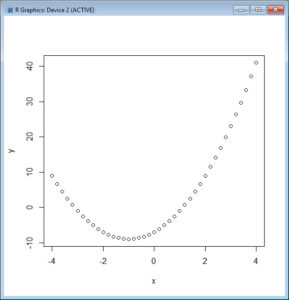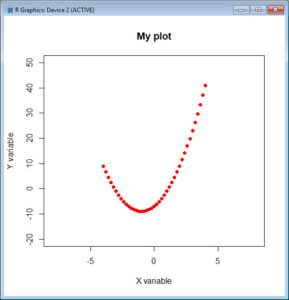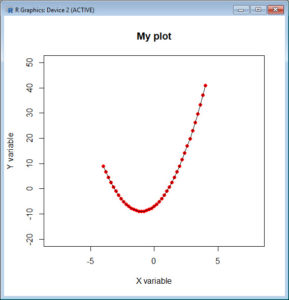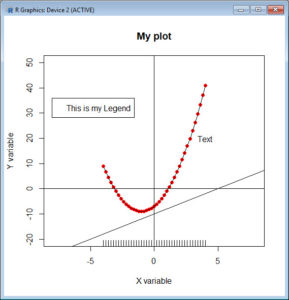In Part 6, let’s look at basic plotting in R. Try entering the following three commands together (the semi-colon allows you to place several commands on the same line).

```x <- seq(-4, 4, 0.2) ;  y <- 2*x^2 + 4*x - 7 plot(x, y)```This is a very basic plot, but we can do much better. Let’s build a nicer plot in several steps.

`plot(x, y, pch = 16, col = "red")`The argument `pch = 16` gave us solid circles, while `col = "red"` coloured those circles red.

Now try:

`plot(x, y, pch = 16, col = "red", xlim = c(-8, 8), ylim = c(-20, 50), main = "My plot", xlab = "X variable" , ylab = "Y variable")`You can see what the `main` argument and the `xlim` and `ylim` arguments achieved. Now try:

```plot(x, y, pch = 16, col = "red", xlim = c(-8, 8), ylim = c(-20, 50), main = "My plot", xlab = "X variable" , ylab = "Y variable") lines(x, y)```Note that the `lines()` command is used after the `plot()` command. Now enter the following syntax.

```abline(h = 0) abline(v = 0) abline(-10, 2)      # Draws a line of intercept -10 and slope 2. text(4, 20, "Text") # Now your text is located at the point (4, 20) on your plot. legend(-8, 36,"This is my Legend")```

Your legend is now centred on the point (-8, 36)

`rug(x)`

The `rug()` command indicates the location of points on the horizontal axis. Here is the final plot:That wasn’t so hard! In blog 7 we will look at some more sophisticated plotting techniques in R.

Good bye for now!

David

#### Annex: R codes used

```# Creating and plotting a function
x <- seq(-4, 4, 0.2); y <- 2*x^2 + 4*x - 7
plot(x, y)

# Building a nicer plot in several steps
# Version 1
plot(x, y, pch = 16, col = "red")

# Version 2
plot(x, y, pch = 16, col = "red", xlim = c(-8, 8), ylim = c(-20, 50), main = "My plot", xlab = "X variable", ylab = "Y variable")

# Version 3
plot(x, y, pch = 16, col = "red", xlim = c(-8, 8), ylim = c(-20, 50), main = "My plot", xlab = "X variable", ylab = "Y variable")
lines(x, y)